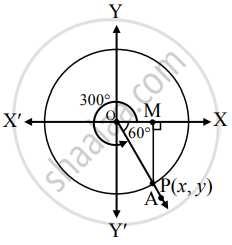# Find the trigonometric function of : 300° - Mathematics and Statistics

Diagram
Sum

Find the trigonometric function of :

300°

#### Solution

Angle of measure 300°:Let m∠XOA = 300°

Its terminal arm (ray OA) intersects the standard unit circle at P(x, y).

Draw seg PM perpendicular to the X-axis.

∴ ΔOMP is a 30° – 60° – 90° triangle.

OP = 1

OM = 1/2"OP"

= 1/2(1)

= 1/2

PM = sqrt(3)/2"OP"

= sqrt(3)/2(1)

= sqrt(3)/2

Since point P lies in the 4th quadrant,

x > 0, y < 0

∴ x = OM = 1/2 and y = -"PM" = (-sqrt(3))/2

∴ P = (1/2, (-sqrt(3))/2)

sin 300° = y = (-sqrt(3))/2

cos 300° = x = 1/2

tan 300° =  = y/x = (-(sqrt(3))/2)/(1/2) = -sqrt(3)

cosec 300° = 1/y = 1/((-(sqrt(3))/2)) = (-2/sqrt(3))

sec 300° = 1/x = 1/((1/2)) = 2

cot 300° = x/y = (1/2)/(-sqrt(3)/2) = -1/sqrt(3)

Concept: Trigonometric Functions of Specific Angles
Is there an error in this question or solution?